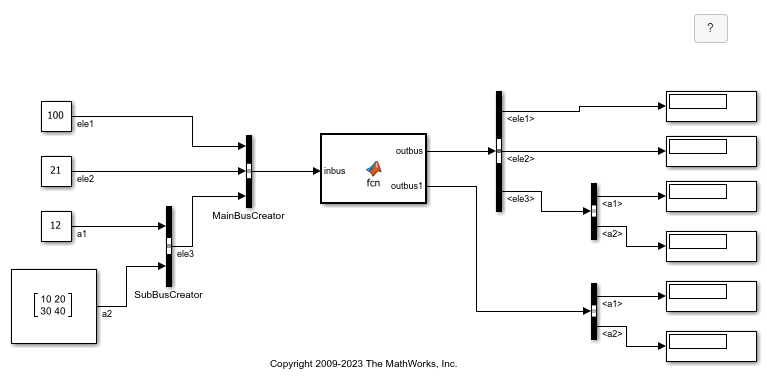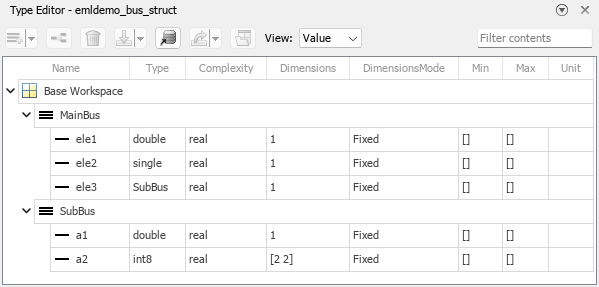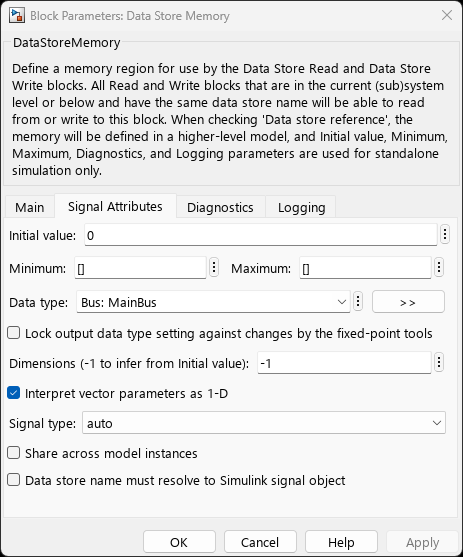## Attach Buses to MATLAB Function Blocks

MATLAB Function can retrieve and output Simulink® buses. When you connect a bus to a MATLAB Function block, the block associates that signal with a structure. For more information on creating structures in MATLAB Function blocks, see Create Structures in MATLAB Function Blocks. You can also retrieve bus data from Data Store Memory blocks. For more information on using buses and Data Store Memory blocks, see Data Stores with Buses and Arrays of Buses.

Note

MATLAB Function blocks convert input virtual buses to nonvirtual buses and output only nonvirtual buses.

### Read and Write Buses with a MATLAB Function Block

This example uses structures in a MATLAB Function block to read and write to Simulink buses.Open the `emldemo_bus_struct` model. The inbus port of the MATLAB Function block connects to a bus and the outbus and outbus1 ports output buses. The input signal comes from the Bus Creator block `MainBusCreator`, which bundles the signals `ele1`, `ele2`, and `ele3`. The signal `ele3` is the output of another Bus Creator block, `SubBusCreator`, which bundles the signals `a1` and `a2`. The outbus and outbus1 ports connect to Bus Selector blocks that, in turn, connect to Display blocks.

`Simulink.Bus` Objects Defined In Simulink

In this example, each structure in the MATLAB Function block must correspond to a `Simulink.Bus` object. Consequentially, the structures in the block share the same properties as the `Simulink.Bus` object they are assigned to, including the number, name, type, and sequence of fields. This model defines two `Simulink.Bus` objects, `MainBus` and `SubBus`. Loading the model loads the buses into the base workspace. You can view the bus definitions and their elements in the Type Editor. In the Modeling tab, in the Design section, click Type Editor. In the Type Editor, set View to `Value`.The model uses these buses in the Bus Creator blocks. `MainBusCreator` outputs a bus with the same type as `MainBus`, and `SubBusCreator` outputs a bus with the same type as `SubBus`.

Structure Definitions in the MATLAB Function Block

Inspect the function `fcn` by opening the MATLAB Function block. The code defines a local variable `mystruct` using the `struct` function, and uses this local variable to initialize the value of the first output, `outbus`. The function initializes the second output, `outbus1`, to the value of the `ele3` field in `inbus`.

```function [outbus, outbus1] = fcn(inbus) ```
```substruct.a1 = inbus.ele3.a1; substruct.a2 = int8([1 2;3 4]); ```
```mystruct = struct('ele1',20.5, 'ele2', single(100), 'ele3', substruct); ```
```outbus = mystruct; outbus.ele3.a2 = 2*(substruct.a2); ```
```outbus1 = inbus.ele3; ```

View the definitions of the structures in the MATLAB Function block. In the Function tab, click Edit Data. Click a variable to see its properties in the Property Inspector. In this example, `inbus` inherits its type, and therefore the Symbols pane does not display the structure elements for `inbus`. By contrast, the Type property of the output variables are set to buses, and the Symbols pane shows the bus elements associated with each variable. Expand the lists of the output structures in the Symbols pane to view the fields.### Write Buses From Data Store Memory to a MATLAB Function Block

This example shows how to use bus data stored in a data store as an input to a MATLAB Function block.Capture the Bus Data in a Data Store Memory Block

In this example, the Bus Creator block `MainBusCreator` creates a bus called `MainBus` because the Output data type parameter is `Bus: MainBus`. The Data Store Write block then uses the Data store name parameter to write the bus data to a data store named `inbus`.

To store the bus data from the Data Store Write block, the model includes a Data Store Memory block. In the Data Store Memory block, the Data store name parameter is `inbus`, the name of the data store defined by the Data Store Write block. The Data type parameter is `Bus: MainBus`, the data type specified by the `MainBusCreator` block.Define the Data Store Memory Variable

To capture the data store in a variable, the MATLAB Function block uses variables that have the Scope property set to `Data Store Memory`. The function then defines the variable as a global variable with the name of the data store, which is `inbus`. Double-click the MATLAB Function block to examine the code.

```function [outbus, outbus1] = fcn ```
```global inbus; ```
```substruct.a1 = inbus.ele3.a1; substruct.a2 = int8([1 2;3 4]); ```
```mystruct = struct('ele1',20.5, 'ele2', single(100), 'ele3', substruct); ```
```outbus = mystruct; outbus.ele3.a2 = 2*(substruct.a2); ```
```outbus1 = inbus.ele3; ```

You can adjust the properties of the data store memory variable in the Symbols pane, Property Inspector, or the Model Explorer.